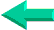### This is an excerpt from Pierre Leclerc (www.excel-vba.com)

The 2 Most Important

Excel Functions

AND
Formulas

SUMPRODUCT

AND

INDEX/MATCH

## Lesson 7: Excel Spreadsheets Lookup and Reference Functions (18)

In annex 7 you have found a description of all 18 Excel functions in the "LookUp and Reference" category. Below is the list of the 5 most useful ones. See more on the very powerful INDEX/MATCH Excel formulas in lesson 12 and in the three old lookup functions in lessons 17,  18 and 19: HLOOKUP function and VLOOKUP function.

 Functions What it Does INDEX Uses an index to choose a value from a reference or array (Powerful in INDEX/MATCH Formulas) MATCH Looks up values in a reference or array (Powerful in INDEX/MATCH Formulas) INDIRECT Returns a reference indicated by a text value OFFSET Returns a reference offset from a given reference ADDRESS Returns a reference as text to a single cell in a worksheet

The most important functions in this category

INDEX, MATCH

See lesson 12 on INDEX/MATCH

The LOOKUP Group

The functions in this group are widely known among  advanced users. But once they discover the more powerful and less limited INDEX/MATCH they are kind of pushed aside. Click on the links below to access the pages of this website describing how they work and what is their limits.

Excel Lesson 17 - Excel HLOOKUP Function
Excel Lesson 18 - Excel LOOKUP Function
Excel Lesson 19 - Excel VLOOKUP Function

Other Functions

When you start developing more complex business models or when you want to calculate and chart moving averages and moving " Year to Date" you will need the two following functions.

INDIRECT
If in cell A1 of Sheet1 you have this value (Sheet2!A1) and in cell A2 of Sheet1 you have the following formula:
=INDIRECT(A1) the result will be the value of  cell A1 of Sheet2.

OFFSET
The most intellectually challenging function in Excel.

The general format of this function goes as follows:
=SUM(OFFSET(D1,1,1,3,3))
In plain English...sum the range of 3 rows by three columns that starts 1 row below and one column to the right of D1 (the anchor). So if you have 2  in all 9 cells E2 to G4 the result will be 18.

Tutorial and Examples

With INDEX and MATCH you can semi automate your invoices so that when you enter the name of a client it's address appears in the cell below and when you enter the product number it's description appears in the cell to the right.

SUMPRODUCT  ¦  INDEX/MATCH  ¦  SUBTOTAL  ¦  ISERROR  ¦  HLOOKUP  ¦  LOOKUP  ¦  VLOOKUP
AVERAGEIF  ¦  AVERAGEIFS ¦  COUNTIF  ¦  COUNTIFS  ¦  SUMIF  ¦  SUMIFS  ¦  IFERRORBack home